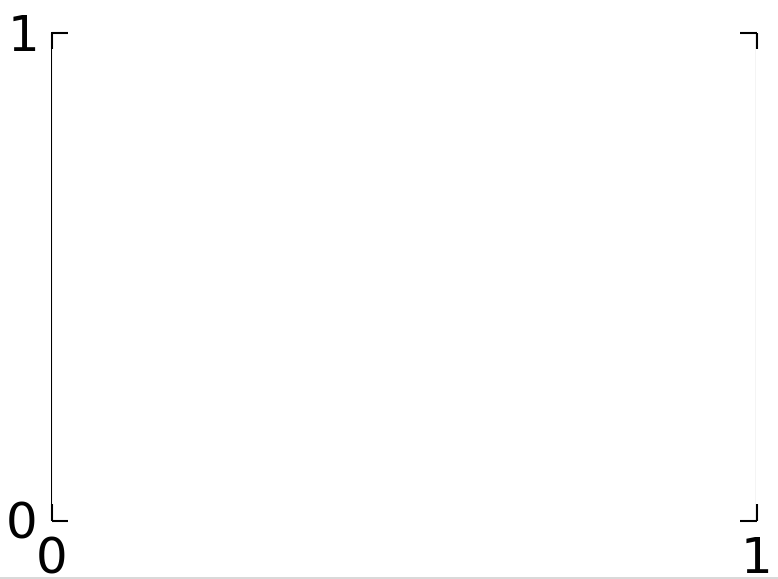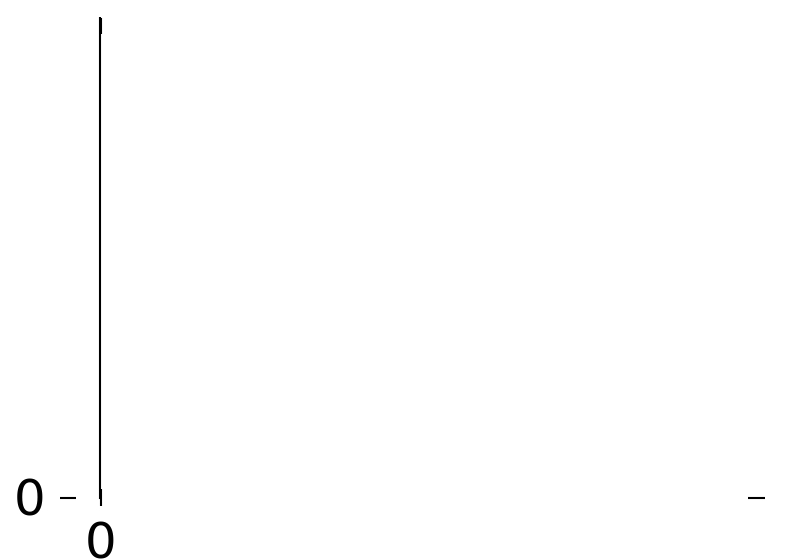# Line2D and ticks are misaligned in TkAgg backend

Hi,

I need to set the frame off for my plots, so that I can have axes only on the sides I want, rather than on all four sides.

I do it this way:

from pylab import *

fig = figure(figsize=(3,2),dpi=300,facecolor=‘w’)
ax =
ax.set_yticks([0,1])
ax.set_xticks([0,1])
show()

Unfortunately, now the ticks and axes-lines are misaligned, as seen in the screenshots attached. In the second screenshot, I’ve panned the plot, so the misalignment is even more visible.

matplotlib.version gives ‘1.1.1rc’

matplotlib.get_backend() gives ‘TkAgg’···

If I use ‘WX’ backend by adding these two line before the above code (before
pylab import):

import matplotlib
matplotlib.use(‘WX’)

then the misalignment still appears to be there, but that is because the Line2Ds are clipped in their width, but the ticks are not. This is seen by panning the plot.

I can set the clipping of the Line2Ds off by doing:

l1 = ax.add_artist(Line2D((0, 0), (0, 1),color=‘k’,linewidth=0.5))
l1.set_clip_on(False)
l2 = ax.add_artist(Line2D((1, 1), (0, 1),color=‘k’,linewidth=0.5))
l2.set_clip_on(False)

So, WX backend is fine.

Backend ‘GTK’ doesn’t even have the above clipping problem.

But any workarounds for the TkAgg backend? Because my production
machine doesn’t have either wx or pygtk installed, so I can only use TkAgg.

Thanks,

Hi,

I need to set the frame off for my plots, so that I can have axes only on the sides I want, rather than on all four sides.

I do it this way:

from pylab import *

fig = figure(figsize=(3,2),dpi=300,facecolor=‘w’)
ax =
ax.set_yticks([0,1])
ax.set_xticks([0,1])

show()

Much easier way is to use “spines”: http://matplotlib.org/examples/pylab_examples/spine_placement_demo.html

Unfortunately, now the ticks and axes-lines are misaligned, as seen in the screenshots attached. In the second screenshot, I’ve panned the plot, so the misalignment is even more visible.

matplotlib.version gives ‘1.1.1rc’

matplotlib.get_backend() gives ‘TkAgg’

If I use ‘WX’ backend by adding these two line before the above code (before
pylab import):

import matplotlib
matplotlib.use(‘WX’)

then the misalignment still appears to be there, but that is because the Line2Ds are clipped in their width, but the ticks are not. This is seen by panning the plot.

I can set the clipping of the Line2Ds off by doing:

l1 = ax.add_artist(Line2D((0, 0), (0, 1),color=‘k’,linewidth=0.5))
l1.set_clip_on(False)
l2 = ax.add_artist(Line2D((1, 1), (0, 1),color=‘k’,linewidth=0.5))
l2.set_clip_on(False)

So, WX backend is fine.

Backend ‘GTK’ doesn’t even have the above clipping problem.

Now that is interesting and should be investigated further.

Ben Root

···

On Thu, Feb 28, 2013 at 7:00 AM, Aditya Gilra <aditya_gilra@…9…> wrote:

Hi Ben,

Thanks for the pointer to “spines”. Much cleaner way to hide/play around with axes. I switched to using spines to hide right and top axes. However, the misalignment of ticks and axes remains (TkAgg backend). So I’ll just have to switch to a different backend I suppose…

···

From: Benjamin Root <ben.root@…3421…>
Cc: "matplotlib-users@lists.sourceforge.net" <matplotlib-users@…431…ists.sourceforge.net>
Sent: Friday, 1 March 2013 12:10 AM
Subject: Re: [Matplotlib-users] Line2D and ticks are misaligned in TkAgg backend

On Thu, Feb 28, 2013 at 7:00 AM, Aditya Gilra <aditya_gilra@…9…> wrote:

Hi,

I need to set the frame off for my plots, so that I can have axes only on the sides I want, rather than on all four sides.

I do it this way:

from pylab import *

fig = figure(figsize=(3,2),dpi=300,facecolor=‘w’)
ax =
ax.set_yticks([0,1])
ax.set_xticks([0,1])

show()

Much easier way is to use “spines”: http://matplotlib.org/examples/pylab_examples/spine_placement_demo.html

Unfortunately, now the ticks and axes-lines are misaligned, as seen in the screenshots attached. In the second screenshot, I’ve panned the plot, so the misalignment is even more visible.

matplotlib.version gives ‘1.1.1rc’

matplotlib.get_backend() gives ‘TkAgg’

If I use ‘WX’ backend by adding these two line before the above code (before
pylab import):

import matplotlib
matplotlib.use(‘WX’)

then the misalignment still appears to be there, but that is because the Line2Ds are clipped in their width, but the ticks are not. This is seen by panning the plot.

I can set the clipping of the Line2Ds off by doing:

l1 = ax.add_artist(Line2D((0, 0), (0, 1),color=‘k’,linewidth=0.5))
l1.set_clip_on(False)
l2 = ax.add_artist(Line2D((1, 1), (0, 1),color=‘k’,linewidth=0.5))
l2.set_clip_on(False)

So, WX backend is fine.

Backend ‘GTK’ doesn’t even have the above clipping problem.

Now that is interesting and should be investigated further.

Ben Root